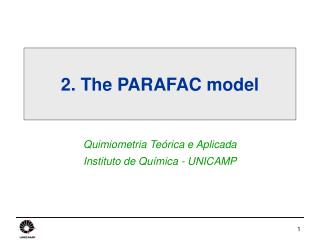DownloadDownload Presentation2. The PARAFAC model

# 2. The PARAFAC model

Download Presentation## 2. The PARAFAC model

- - - - - - - - - - - - - - - - - - - - - - - - - - - E N D - - - - - - - - - - - - - - - - - - - - - - - - - - -
##### Presentation Transcript

1. 2. The PARAFAC model Quimiometria Teórica e Aplicada Instituto de Química - UNICAMP

2. Example: fluorescence data (1) Each fluorescence spectrum is a matrix of emission vs excitation wavelengths: Xi (201  61)

3. emission spectrum of pure tryptophan concentration of tryptophan in sample i excitation spectrum of pure tryptophan c3T c1T c2T Xi ai3  ai1  ai2  = + + b3 b1 b2 Example: fluorescence data (2) • Each spectrum is a linear sum of three components: tryptophan, phenylalanine and tyrosine. Xi = ai1b1c1T+ai2b2c2T+ ai3b3c3T + Ei +Ei

4. c2T c3T c1T X5 b2T b3T b1T X4 X1 = + X3 + X2 5 samples 61 excitation ’s a2 a3 a1 201 emission ’s concentration of tryptophan in each sample Example: fluorescence data (3) • Five samples were measured and stacked to give a three-way array: X (5  201  61). + E

5. Example: fluorescence data (4) • If we are given a set of fluroescence spectra, X, how can we determine: • How many chemical species are present? • Which chemical species are present? What are their pure excitation and emission spectra? • i.e. self-modelling curve resolution (SMCR) • What is the concentration of each species in each sample? • i.e. (second-order) calibration • Answer: use the PARAFAC model!

6. c1T c2T cRT + … + + E b1T b2T bRT X a1 a2 aR K I } J Triad CT = + BT E A The PARAFAC model (1) =

7. CT = + BT E A The PARAFAC model (2) X K I J • Loadings • A (IR) describes variation in the first mode. • B (JR) describes variation in the second mode. • C (KR) describes variation in the third mode. • Residuals • E (IJK) are the model residuals.

8. CT = + BT E 5 samples 61 excitation ’s 201 emission ’s A Example: fluorescence data (5) X • Loadings • A (5  3) describes the component concentrations. • B (201  3) describes the pure component emission spectra. • C (61  3) describes the pure component excitation spectra. • Residuals • E (5  201  61) describes instrument noise.

9. B (201  3) C (61  3) phenylalanine phenylalanine tyrosine tyrosine tryptophan tryptophan Example: fluorescence data (6) • A 3-component PARAFAC model describes 99.94% of X.

10. A (5  3) -0.0853 -1.8151 2.7867 -0.0135 -0.0042 13.172 0.2714 0.0147 2.0803 0.0006 0.1484 785.09 0.0492 0.0234 1.8358 5.3045 341.68 1.6140 0.8378 0.7990 0.8790 4.4000 297.00 0.9179 0.6949 0.6945 Example: fluorescence data (7) • The A-loadings describe the relative amounts of species 1 (tryptophan), 2 (tyrosine) and 3 (phenylalanine) in each sample: Concentrations (ppm) 2.6685 0.0141 0.0471 1.5455 • In order to know the absolute amounts, it is necessary to use a standard of known concentrations, i.e. sample 5.

11. Khatri-Rao matrix product The PARAFAC formula • Data array • X (IJK) is matricized into XIJK (IJK) XIJK = A(CB)T + EIJK • Loadings • A (IR) describes variation in the first mode • B (JR) describes variation in the second mode • C (KR) describes variation in the third mode • Residuals • E (IJK) is matricized into EIJK (IJK)

12. Trilinear model Bilinear model XIJK = A(CB)T + EIJK X = ABT + E PCA vs PARAFAC PCA PARAFAC Components are calculated sequentially in order of importance. Components are calculated simultaneously in random order. Orthogonal, i.e.BTB = I Not (usually) orthgonal. Solution has rotational freedom. Solution is unique (i.e. not possible to rotate factors without losing fit).

13. Rotational freedom • The bilinear model X = ABT + E contains rotational freedom. There are many sets of loadings (and scores) which give exactly the same residuals, E: X = ABT+ E = ARR-1BT+ E = A*B*T+ E (A*=AR B*T=R-1BT) • This model is not unique – there are many different sets of loadings which give the same % fit.

14. PARAFAC solution is unique • The trilinear model X= A(CB)T + E is said to be unique, because it is not possible to rotate the loadings without changing the residuals, E: X = A(CB)T + E = ARR-1(CB)T + E = A*(C*B*)T + E* • This is why PARAFAC is able to find the correct fluorescence profiles – because the unique solution is close to the true solution.